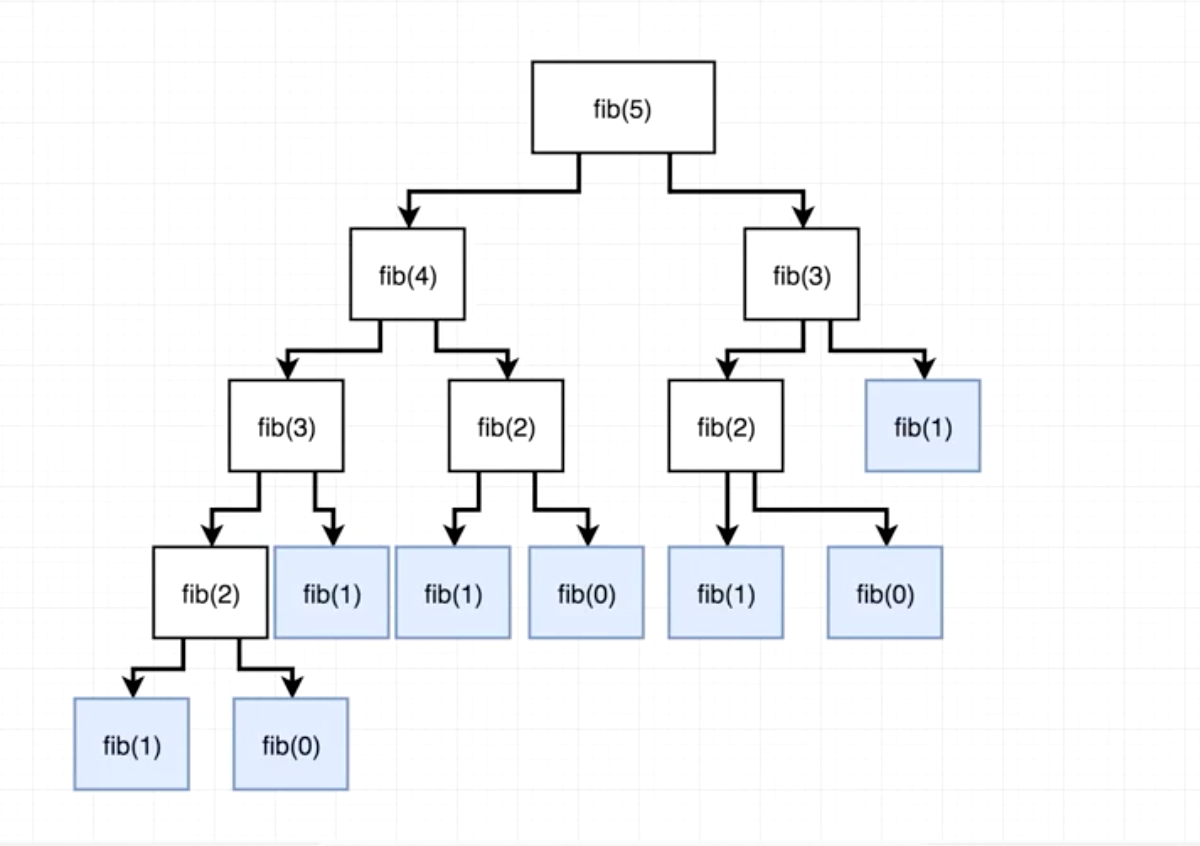# Fibonacci sequence JavaScript interview question. Iterative and Recursive solutions.

“Write a function to return an n element in Fibonacci sequence” is one of the most common questions you can hear during the coding challenge interview part. In this blogpost I’m going to walk through the two of the most typical solutions for this problem and also cover a dreadful (for most of novice developers) topic of time complexity.

So what is a Fibonacci sequence? According to Wikipedia:

“In mathematics, the Fibonacci numbers are the numbers in the following integer sequence, called the Fibonacci sequence, and characterized by the fact that every number after the first two is the sum of the two preceding ones.”

Depending on the chosen starting point of the sequence (0 or 1) the sequence would look like this:

1, 1, 2, 3, 5, 8, 13, 21, 34, 55, 89, 144, …

or this:

0, 1, 1, 2, 3, 5, 8, 13, 21, 34, 55, 89, 144, …

FUN FACT: Fibonacci sequence, also known as the Golden Ratio, appears a lot in nature. Patterns such as spirals of shells, curve of waves, seed heads, pinecones, and branches of trees can all be described using this mathematical sequence. The fact that things as large as spirals of galaxies, and as small as DNA molecules follow the Golden Ratio rule suggests that Fibonacci sequence is one of the most fundamental characteristics of the Universe.

Alright, now back to Earth and our Fibonacci sequence coding challenge. Let’s quickly describe a test case for our fib() function. If we were to take a short Fibonacci sequence: [0, 1, 1, 2, 3, 5, 8, 13, 21] and fib(4), the result would be equal to 3, so basically we need to return an element with index 4 from our Fibonacci sequence array.

One possible and probably the easiest solution that comes to mind here is iteration. Let’s see how it would look:

`function fib(n){  let arr = [0, 1];  for (let i = 2; i < n + 1; i++){    arr.push(arr[i - 2] + arr[i -1])  } return arr[n]}`

So notice that two first numbers can not really be effectively generated by a for loop, because our loop will involve adding two numbers together, so instead of creating an empty array we assign our arr variable to [0, 1] that we know for a fact will always be there. After that we create a loop that starts iterating from i = 2 and adds numbers to the array until the length of the array is equal to n + 1. Finally, we return the number at n index of array.

`fib(4)=> 3`

Great, seems like this works. Now what if your interviewer thinks this is not enough and asks you to implement a recursive solution?

Although recursive solution looks pretty simple it is pretty tricky to arrive to if you’ve never previously encountered it:

`function fib(n) {  if (n < 2){    return n  }  return fib(n - 1) + fib (n - 2)}`

So, our base case here is returning n if it’s value is less that 2. Let’s look at the diagram that will help you understand what’s going on here with the rest of our code. Function fib is called with argument 5:(Diagram from Stephen Grider’s “The Coding Interview Bootcamp“ course on Udemy.com)

Basically our fib function will continue to recursively call itself creating more and more branches of the tree until it hits the base case, from which it will start summing up each branch’s return values bottom up, until it finally sums them all up and returns an integer equal to 5. It might take a moment to sink in, so take some time to look at the tree and you will understand what’s happening there.

For the Fibonacci sequence, it is highly recommended to learn JavaScript. Now that we covered these two common solutions for the problem, let’s see talk about time complexity.

Some testing environments, like Jest for instance indicate how long it took to fun your function in milliseconds. Assuming we had some tests prewritten for this challenge, this is what the results would look for:

Iterative solution:

Recursive solution:

Now look at the case when we call fib() with n=15. It took iterative solution 4ms, but it took recursive solution 1328ms to perform the same action. Why is that?

An algorithm in our iterative solution takes linear time to complete the task. Basically we iterate through the loop n-2 times, so Big O (notation used to describe our worst case scenario) would be simply equal to n in this case.

In case of recursion the solution takes exponential time, that can be explained by the fact that the size of the tree exponentially grows when n increases. So for every additional element in the Fibonacci sequence we get an increase in function calls. Big O in this case is equal to 2^n.

Hopefully now that you conquered Fibonacci sequence coding challenge, you have increased your chances of successfully passing the interview. In the next blogpost I’m going to cover implementation of a possible improvement of recursive solution using memoization. Stay tuned!

--

--

--

## More from Quick Code

Find the best tutorials and courses for the web, mobile, chatbot, AR/VR development, database management, data science, web design and cryptocurrency. Practice in JavaScript, Java, Python, R, Android, Swift, Objective-C, React, Node Js, Ember, C++, SQL & more.

## Enable Cloud Native Service Ownership## Filling holes in 3D mesh using Meshlab## How to Interview a Web Developer and What to Look For?## A Year in the Life of a Software Engineering Intern## Working With Big O Notation## C++ Smart Pointers and how to write your own## 7 Reasons to run React JS for speed boosting in software development vs Angular JS## React , Angular , How to chose which one is the best for you ?## Add key-value pair to every object in array of objects in JavaScript## Searching Algorithms in JavaScript